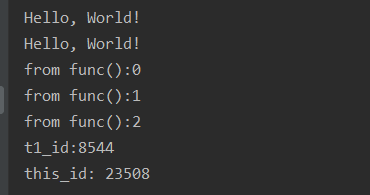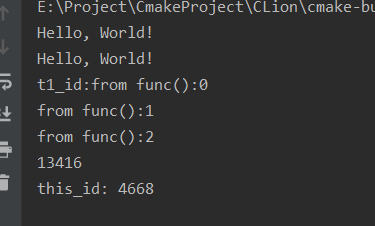# 1. 多线程编程

Qt多线程编程

(1)、get_id：获取线程ID，返回一个类型为std:🧵:id的对象。

(4)、detach：将当前线程对象所代表的执行实例与该线程对象分离，使得线程的执行可以单独进行。一旦线程执行完毕，它所分配的资源将会被释放。

(6)、swap：交换两个线程对象所代表的底层句柄。

(8)、hardware_concurrency：静态成员函数，返回当前计算机最大的硬件并发线程数目。基本上可以视为处理器的核心数目。

## 2.1 创建一个线程

#include <iostream>
#include <stdlib.h> //sleep

using namespace std;

void t1()
{
for (int i = 0; i < 10; ++i)
{
cout << "博客园-进击的汪sir\n";
sleep(1);
}
}
void t2()
{
for (int i = 0; i < 20; ++i)
{
cout << "1111111111111\n";
sleep(1);
}
}
int main()
{

th1.join(); // 必须将线程join或者detach 等待子线程结束主进程才可以退出
th2.join();

//or use detach
//th1.detach();
//th2.detach();

cout << "here is main\n\n";

return 0;
}


## 2.2 lambda表达式

std::thread t3([](int a, double b){cout << a << ' ' << b << endl;}, 3, 4);


# 3. join与detach的区别

## 3.1 join()

join()函数是一个等待线程完成函数，主线程需要等待子线程运行结束了才可以结束

#include <iostream>
using namespace std;
void func()
{
for(int i = 0; i <3; i++)
{
cout << "from func():" << i << endl;
}
}

int main() {
std::cout << "Hello, World!" << std::endl;
std::cout << "Hello, World!" << std::endl;
t1.join();

std::cout << "t1_id:" << t1_id << endl;
std::cout << "this_id: " << this_id <<endl;
return 0;
}## 3.2 detach()

#include <iostream>
using namespace std;
void func()
{
for(int i = 0; i <3; i++)
{
cout << "from func():" << i << endl;
}
}

int main() {
std::cout << "Hello, World!" << std::endl;
t1.detach();
std::cout << "Hello, World!" << std::endl;
std::cout << "t1_id:" << t1_id << endl;
std::cout << "this_id: " << this_id <<endl;
return 0;
}# 4. 参考

https://blog.csdn.net/sevenjoin/article/details/82187127

posted @ 2021-09-10 18:00  进击的汪sir  阅读(85)  评论(0编辑  收藏  举报